Next: Optimisation of the Nudged Up: FINDING REARRANGEMENT PATHWAYS Previous: Introduction   Contents

# A Double-ended Method: Nudged Elastic Band

In the present work we used the nudged elastic band [27,28] (NEB) and eigenvector-following [17,18,12,14,24,13,19,16,22,23,15] (EF) methods for locating and refining transition states. In the NEB approach the path is represented as a set of images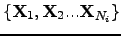that connect the endpoints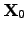and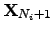,where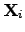is a vector containing the coordinates of image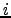(Figure 2.1) . In the usual framework of double-ended methodologies  the endpoints are stationary points on the PES (usually minima), which are known in advance. In addition to the true potential,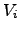, which binds the atoms within each image, equivalent atoms in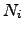adjacent images are interconnected by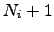springs according to a parabolic potential,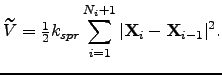(4.1)

Subsequently these potentials will be referred to as the true potential' and the spring potential', respectively.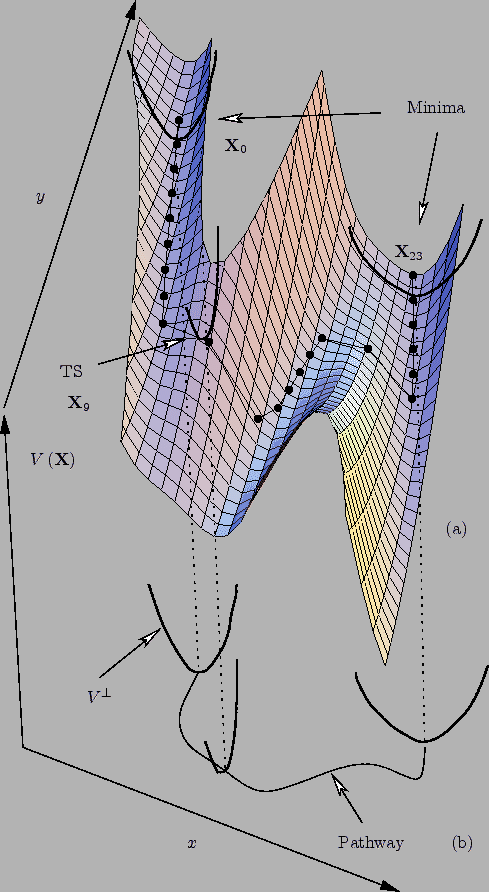The springs are intended to hold images on the path during optimisation -- otherwise they would slide down to the endpoints, or to other intermediate minima . Occasionally, depending on the quality of the initial guess, we have found that some images may converge to higher index stationary points. One could imagine the whole construction as a band or rope that is stretched across the PES, which, if optimised, is capable of closely following a curve defined in terms of successive minima, transition states, and the intervening steepest-descent paths.

In practice, the above formulation encounters difficulties connected with the coupling between the true' and spring' components of the potential. The magnitude of the springs' interference with the true potential is system dependent and generally gives rise to corner-cutting and sliding-down problems . It is convenient to discuss these difficulties in terms of the components of the true gradient,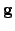, and spring gradient,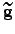, parallel and perpendicular to the path. The parallel component of the gradient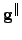at imageon the path is obtained by projecting out the perpendicular component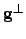using an estimate of the tangent to the path. The parallel and perpendicular components for imageare: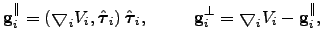(4.2)

where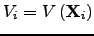, the unit vector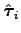is the tangent, and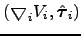denotes the scalar product of vectors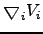and. Here and throughout this work we denote unit vectors by a hat.The complete gradient,, has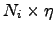components for a band ofimages with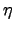atomic degrees of freedom each.

Corner-cutting has a significant effect when a path experiences high curvature. Here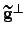is large, which prevents the images from following the path closely because the spring force necessarily has a significant component perpendicular to the tangent. The sliding-down problem occurs due to the presence of, which perturbs the distribution of images along the path, creating high-resolution regions (around the local minima) and low-resolution regions (near the transition states) . Both problems significantly affect the ability of the NEB method to produce good transition state candidates. We have found that sliding-down and corner-cutting are interdependent and cannot both be remedied by adjusting the spring force constant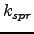;increasingmay prevent sliding-down but it will make corner-cutting worse.

The aforementioned problems can sometimes be eliminated by constructing the NEB gradient from the potential in the following way:andare projected out, which gives the elastic band its nudged' property . Removal ofcan be thought of as bringing the path into a plane or flattening the PES [Figure 2.1 (b)], while removal ofis analogous to making the images heavier so that they favour the bottom of the valley at all times.

The choice of a method to estimate the tangent to the path is important for it affects the convergence of the NEB calculation. Originally, the tangent vector,, for imagewas obtained by normalising the line segment between the two adjacent images,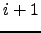and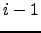: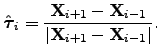(4.3)

However, kinks can develop during optimisation of the image chain using this definition of. It has been shown  that kinks are likely to appear in the regions where the ratio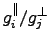is larger than the length of the line segment,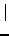, used in estimating the tangent [Figure 2.2 (a)].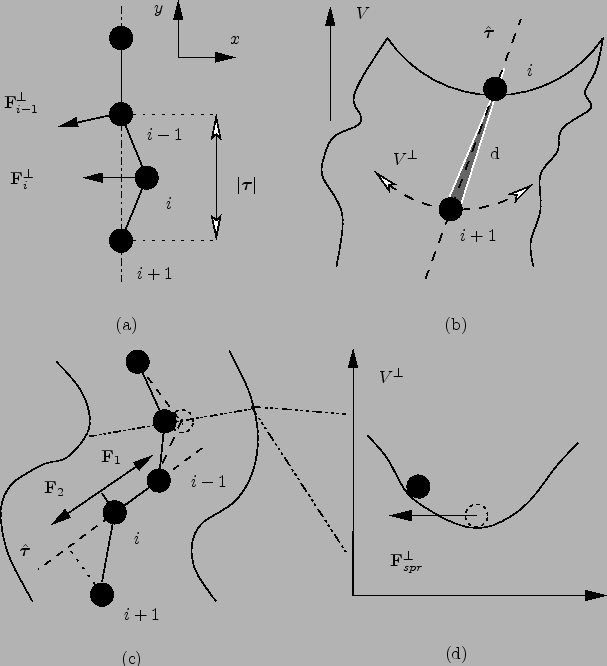Both the above ratio, the image density and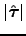can vary depending on the system of interest, the particular pathway and other parameters of the NEB calculation. From Equation 2.3 it can be seen that, and, hence, the next step in the optimisation of image, is determined by its neighbours, which are not necessarily closer to the path than image. Therefore, a better approach in estimating thewould be to use only one neighbour, since then we only need this neighbour to be better converged than image.

There are two neighbours to select from, and it is natural to use the higher-energy one for this purpose, since steepest-descent paths are easier to follow downhill than uphill: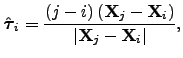(4.4)

whereand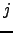are two adjacent images with energiesand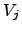, and. In this way, an imagethat has one higher-energy neighbourbehaves as if it is hanging' on to it [Figure 2.2(b)].

The above tangent formulation requires special handling of extrema along the path, and a mechanism for switching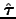at such points was proposed . It also fails to produce an even distribution of images in regions with high curvature [Figure 2.2 (c)]. We presume that Henkelman and Jónsson substitute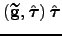by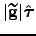in Equation 2.2 to obtain a spring gradient formulation that will keep the images equispaced when the tangent from Equation 2.4 is used in the projections :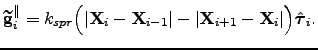(4.5)Next: Optimisation of the Nudged Up: FINDING REARRANGEMENT PATHWAYS Previous: Introduction   Contents
Semen A Trygubenko 2006-04-10